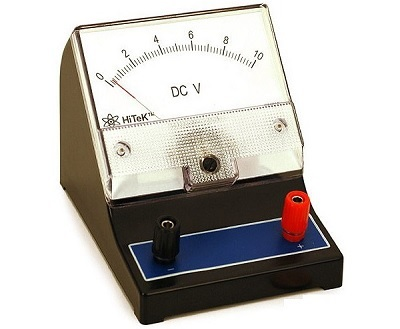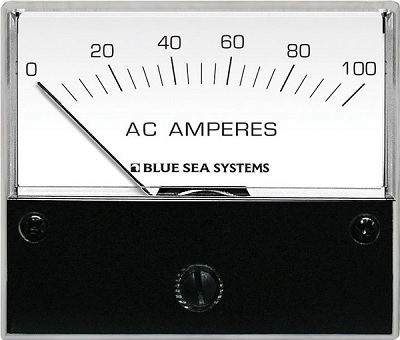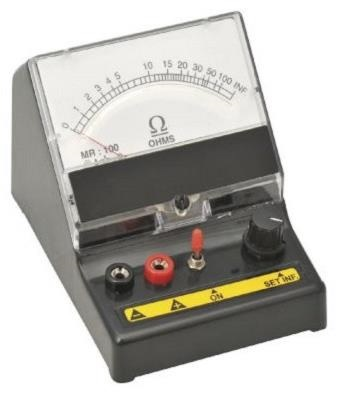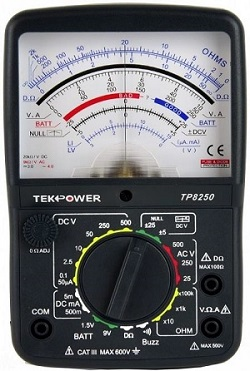# What is Electrical Instruments ?

The instruments, which is use to measure any quantity are known as measuring instruments. This tutorial covers mainly the electronic instruments, which are useful for measuring either electrical quantities or parameters.

Following are the most commonly use electrical instruments.

1. Volt meter
2. Am meter
3. Ohm meter
4. Multi meter

Now, let us discuss about these instruments briefly.

## Voltmeter

As the name suggests, voltmeter is a measuring instrument which measures the voltage across any two points of an electric circuit. There are two types of voltmeters: DC voltmeter, and AC voltmeter.

DC voltmeter measures the DC voltage across any two points of an electric circuit, whereas AC voltmeter measures the AC voltage across any two points of an electric circuit.The DC voltmeter shown in above figure is a (0−100)V DC voltmeter. Hence, it can be used to measure the DC voltages from zero volts to 10 volts.

## Ammeter

As the name suggests, ammeter is a measuring instrument which measures the current flowing through any two points of an electric circuit. There are two types of ammeters: DC ammeter, and AC ammeter.

DC ammeter measures the DC current that flows through any two points of an electric circuit. Whereas, AC ammeter measures the AC current that flows through any two points of an electric circuit.The AC ammeter shown in above figure is a (0−100)A AC ammeter. Hence, it can be used to measure the AC currents from zero Amperes to 100 Ampere.

## Ohm Meter

Ohm meter works on the basis of Ohm’s law.it  is use to measure the value of resistance between any two points of an electrical circuit. Moreover, It also be use for find the value of an unknown resistor. There are two types of ohmmeters: series ohmmeter, and shunt ohm meter.

In series type ohmmeter, the resistor whose value is unknown and to be measure should be connect in series with the ohmmeter. It is useful for measuring high values of resistances.In shunt type ohmmeter, the resistor whose value is unknown and to be measured should be connected in parallel (shunt) with the ohmmeter. It is useful for measuring low values of resistances.An example of practical shunt ohm Meter is shown in the above figure. The ohmmeter shown in above figure is a (0−100)ΩΩ shunt ohm Meter. Hence, it can be used to measure the resistance values from zero ohms to 100 ohms.

### Multi Meter

Multi meter is an electronic device which is use to measure the electrical quantity such as voltage, current & resistance one at a time. It is use to measure DC & AC voltages. DC & AC currents and resistance of several ranges.As shown in the figure, this multi meter can be used to measure various high resistances, low resistances, DC voltages, AC voltages, DC currents, & AC currents. Different scales and range of values for each of these quantities are marked in above figure.The instruments which we considered in this chapter are of indicating type instruments, as the pointers of these instruments deflect and point to a particular value. We will discuss about these electronic measuring instruments in detail in later chapters.

## 2 Comments to “What is Electrical Instruments ?”

1.Ntl

Good Topic

2.Holli baugh

This is perfect for Electrical Studies